# Struct and functions when using the Arduino IDE

As anyone reading this blog probably knows, the Arduino IDE simplifies a number of programming for an embedded environment and hides some of the required C / C++ material. This can make life a lot easier, but it can also cause problems, especially when you step out to do more complex things. I got bit by one of those earlier today. Since I eventually found a post to the work around, I thought I’d post it here.

In my robot code, I”ve defined a struct called coord that holds two doubles, which are the x and y coordinates for whatever I need (e.g., the position of the robot, the next waypoint, etc.

Today, I wanted to compute the distance from the ray defined by the previous and next waypoint and the current position of the vehicle, so that the error could be fed into a PID controller. I figured it would be easy to pass the parameters as coord types. BUT, this turns out to be trickier than it should be with the Arduino. Unless the structs are defined in a .h file, there are problems with their scope. A work-around is documented by Alexander Brevig on the Arduino Playground: Struct Resource for Arduino.

# A Simple Low-Pass Filter (Concluded)

The last post covered the concept of the Exponentially Weighted Moving Average Filter and illustrated how it worked on a theoretical example, both with and without noise.  To wrap up, I want to include an actual set of data from the Devantech CMPS10 Tilt Compensated Compass on my current robot.  Although, as the name says, it has tilt compensation, I’m using the raw magnetometer output, as the robot is running on flat floors, and rotations and accelerations are liable to introduce more error than the practically non-existent tilt.

In my code, I’ve set α to a fairly high value of 0.33.  Here’s a plot of both the raw and filtered output for a case where the robot stayed fixed, then was manually rotated rather quickly to a new position: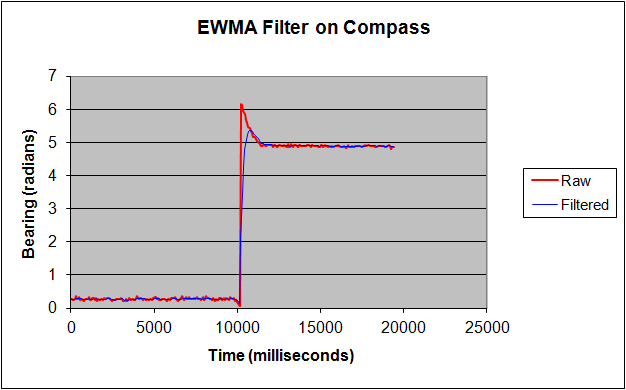As you can see form the plot, the filtered response, as expected, lags the raw response after the turn.  However, the raw output overshoots (I’m not sure why), so the lag actually results in the filtered output more closely matching reality, even right after the turn.  It’s not possible to see the effects of the filter on the noise from this plot, so this second graph shows just the raw and filtered data before the rotation, with the scale blown up: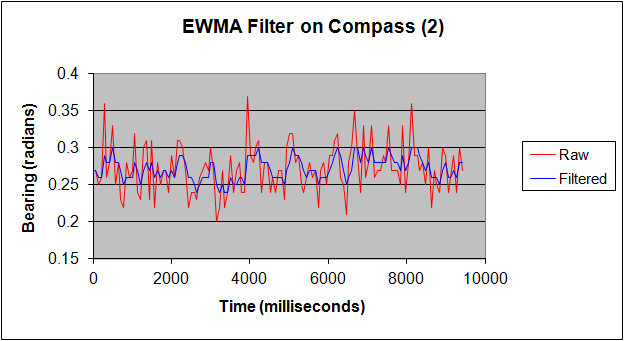The filter’s ability to modulate the noise is clearly evident.  The raw output bounces around +/- about 4.6 degrees, while the filtered output jumps within the much narrower range of +/- 1.5 degrees.

# Filtering Noise from Sensor Readings: A Simple Low Pass Filter

In a perfect world, a sensor’s output would exactly match the input it is sensing.  In the real world, there are a number of errors introduced, including bias, time lags in response, and noise.  This article discusses one of the simplest filters for reducing noise: the Exponentially Weighted Moving Average (EWMA) filter.  Despite its long name, it’s about the simplest and most efficient filter you can implement.  The name comes from the concept that the filter’s output, y, is the weighted average of the current input and each previous inputs, with the weighting decreasing exponentially:

Yt = α (Xt + (1 – α) Xt-1 + (1-α)2 Xt-2 + (1-α)3 Xt-3 + ….

where Yt is the current output from the filter, and Xt is the current input, and the other X’s are each preceding value.  The weighting is shown in the figure: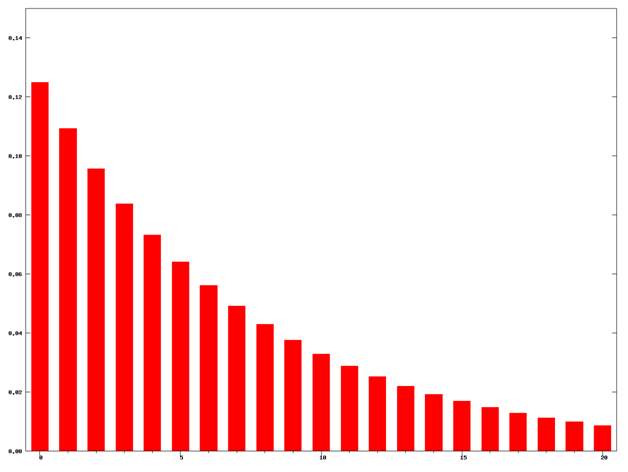This averaging tends to smooth out the response and reduce noise.  Of course, this formula would be cumbersome and time consuming to implement in an embedded system.  Even if only a small number of past values were used.  Some math can show that the formula can be rewritten as a recursive formula using the past output from the filter:

Yt = α Yt-1  + (1 – α) Xt for all values of t > 0, or, re-arranging terms:

Yt =  Xt  + α ( Yt-1 – Xt)

This means that all you need to store is the immediate past output of the filter, which already has the weighted average of all previous values calculated in. This is simpler and takes less storage than, for example, averaging the current and past 4 values of the sensor.  It will also respond more quickly to actual changes that you are trying to measure.

## Limits

You can choose a value for α ranging between 0 and 1.  At 0, the output is just the raw input: no filtering occurs.  The output will respond instantly both to noise and to true changes in the system.

At 1, the output becomes equal to the past value: it never changes, eliminating all noise and all real signal changes!  Clearly one wants to select a value in between these extremes.

## Selecting α

The correct value will depend on your situation.  The higher the a, the more noise if filtered out, but also the more lag that is introduced in reacting to real system changes.  This is shown in the following figures: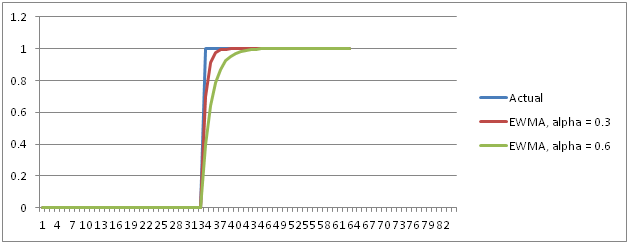This figure shows the response of two filters to a unit step function.  The red curve is for α = 0.3, and the green curve is forα = 0.6.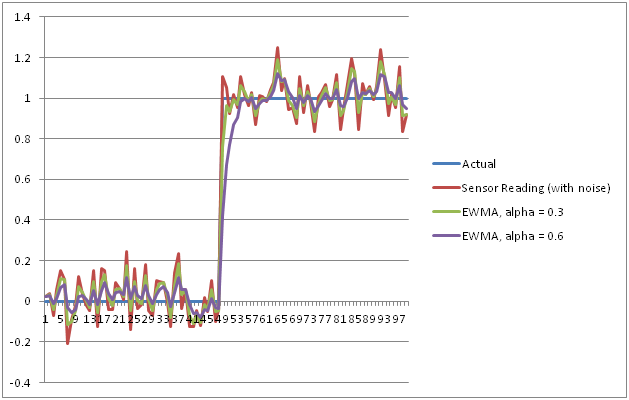This graph shows the output of the filters to a noisy sensor, with Gaussian noise with a standard deviation of 0.1.  One can see from the graph that a value of 0.3 reduces the noise somewhat, but leaves a lot in.  A value of 0.6 greatly reduces the noise, at the expense of greater lag.

## Initializing the Filter

The formulas work for all values after the initial run of the filter.  Like any recursive formula there needs to be a starting value.  My rather rough and informal scanning of the literature identified three common methods:

• For the first pass, set the output equal to the input (same as no filtering)
• Take a small number of readings, e.g.,  5, and use a straight average of these as the initial output.   This is what I did for compass readings in my current robotic vehicle.
• For control processes, an alternative is to set the initial output at the desired control point (figuring, I suppose, that if the control system is working, the average should be at the control point).

## Summary

The simple EWMA routing can be summarized as:

For t= 0:

Yt = Xt

Else:

Yt =  Xt  + α ( Yt-1 – Xt)